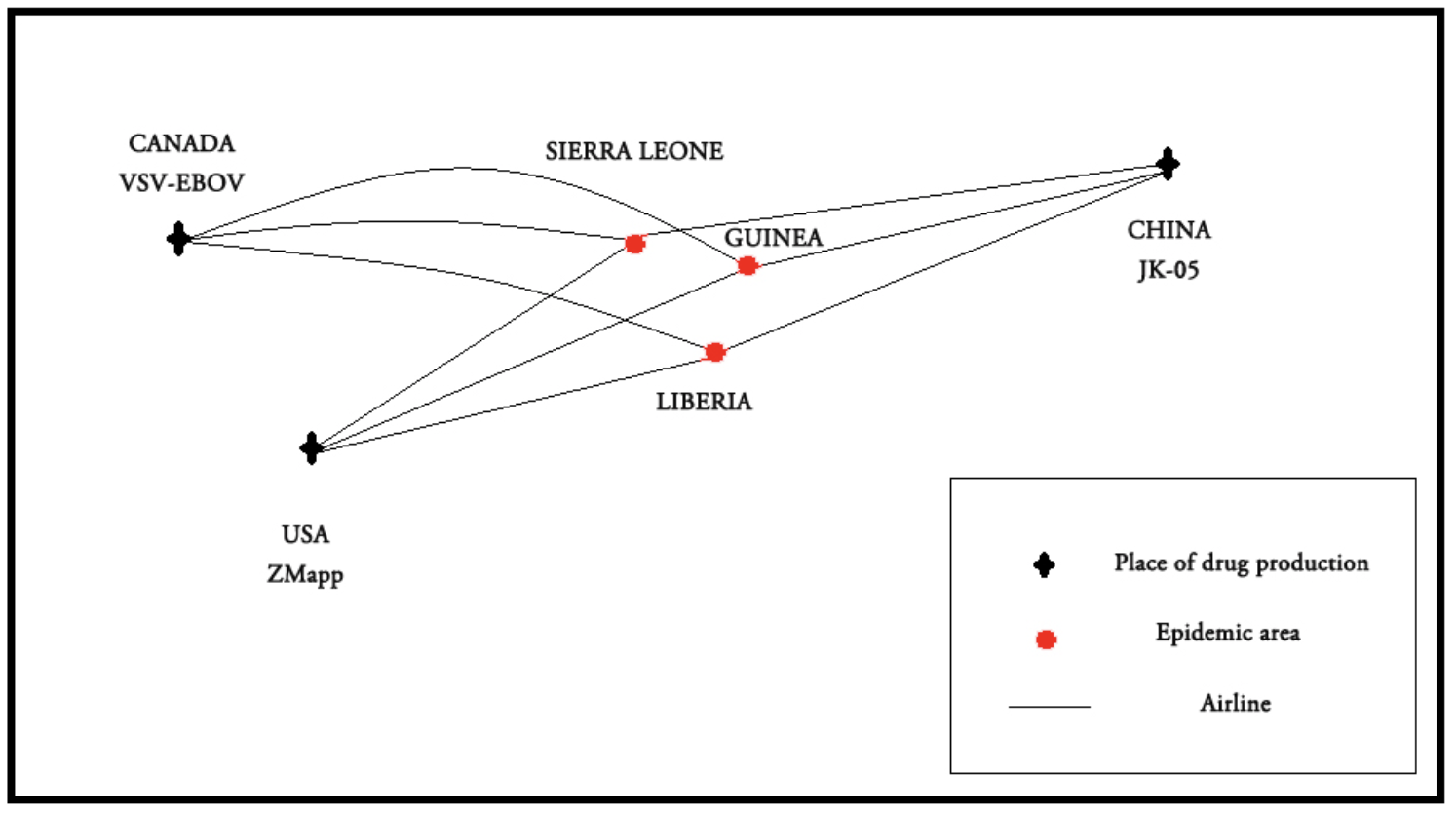# A Numerical Model to Simulate Ebola Epidemic

Published:In Mathematical Contest in Modeling (MCM/ICM) 2015, we chose the problem A. In this problem, we are required to build a mathematical model to describe the process of curbing Ebola, which includes critical factors such as the spread of disease and the distribution of medicine.

Based on famous SEIR model, we build an epidemic region model taking into account the “contact-infection” principle and the variance of resistance among individuals. The refined version also considers the social circle of a person. Simulation results are compared with true data in two African countries to verify its correctness.

## Implementation

• C++ : Basic model
• Python : Refined model

Paper